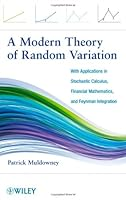# A Modern Theory of Random Variation1 Reviews
2012-10-23
544 pages

## Book Description

A Modern Theory of Random Variation: With Applications in Stochastic Calculus, Mathematics, and Feynman Integration

A ground-breaking and practical treatment of probability and stochastic processes

A Modern Theory of Random Variation is a new and radical re-formulation of the mathematical underpinnings of subjects as diverse as investment, , and mechanics. Setting aside the classical theory of probability measure spaces, the book utilizes a mathematically rigorous version of the theory of random variation that bases itself exclusively on finitely additive probability functions.

In place of twentieth century Lebesgue integration and measure theory, the author uses the simpler concept of Riemann sums, and the non-absolute Riemann-type integration of Henstock. Readers are supplied with an accessible approach to standard of probability theory such as the central limmit theorem and Brownian motion as well as remarkable, new results on Feynman diagrams and stochastic integrals.

Throughout the book, detailed numerical demonstrations accompany the discussions of abstract mathematical theory, from the simplest elements of the subject to the most complex. In addition, an array of numerical examples and vivid illustrations showcase how the presented methods and applications can be undertaken at various levels of complexity.

A Modern Theory of Random Variation is a suitable book for courses on mathematical analysis, probability theory, and mathematical finance at the upper-undergraduate and graduate levels. The book is also an indispensible resource for researchers and practitioners who are seeking new concepts, techniques and methodologies in data analysis, numerical calculation, and financial asset valuation.

Patrick Muldowney, PhD, served as lecturer at the Magee Business School of the UNiversity of Ulster for over twenty years. Dr. Muldowney has published extensively in his areas of research, including integration theory, financial mathematics, and random variation.

1 Prologue 1
2 Introduction 37
3 Infinite-Dimensional Integration 83
4 Theory of the Integral 111
5 Random Variability 183
6 Gaussian Integrals 257
7 Brownian Motion 305
8 Stochastic Integration 383
9 Numerical Calculation 447
A Epilogue 491

## Book Details

• Title: A Modern Theory of Random Variation
• Author:
• Length: 544 pages
• Edition: 1
• Language: English
• Publisher:
• Publication Date: 2012-10-23
• ISBN-10: 111816640X
• ISBN-13: 9781118166406